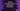# Python Set pop() method explanation with example## Introduction :

Python set pop method is used to remove an element from a set and returns it. It removes a random element. For example :

``set = {1,2,3,4,5}``

If we use pop method, it will remove any random element from this set. The new set will not contain this element after pop is called.

### Syntax of python set pop() :

The syntax of the pop method is as below :

``set.pop()``

As you can see, this method doesn’t take any parameter. It will return one random element from the set. This element is also removed from the set. So, the new set will not contain the returned element.

``````setA = {1, 2, 3, 4, 5, 6, 7, 8, 9}

print("setA : {}".format(setA))
print("The popped item is : {}".format(setA.pop()))
print("setA : {}".format(setA))``````

### Sample Output :

``````setA : {1, 2, 3, 4, 5, 6, 7, 8, 9}
The popped item is : 1
setA : {2, 3, 4, 5, 6, 7, 8, 9}``````

If the set is empty, it will throw one TypeError.

``````setA = set()

print("The popped item is : {}".format(setA.pop()))``````

It will throw one TypeError.

``TypeError: pop expected at least 1 arguments, got 0``